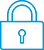cancel
Showing results for
Search instead for
Did you mean:It has been a while since anyone has replied. Simply ask a new question if you would like to start the discussion again.Level 2
16 9 0 0
Message 1 of 1
389
Flag Post

# HP Prime G2 seems not to have any way to show both x & slope value at same time in graphing.HP Recommended
HP Prime calculator
Other

Both the HP Prime G2 and an older version of Prime calculator apparently can show both the x (independent variable) value and y (dependent variable--function) value at the cursor location at the same time (on the same screen) with a function's graph, but not the x value and the slope (derivative wrt x) at that x value--one much switch between graph Fcn option 5 to show slope value, and option 8 (which shows the tangent line and the x & y values but doesn't give the slope value) or Trace to get the x value. This is an obvious problem to (easily, I imagine) correct (unless there is already a way to get both the x-value and the function's derivative at that point on the graph screen at the same time--is there?)

Tags (3)
† The opinions expressed above are the personal opinions of the authors, not of HP. By using this site, you accept the Terms of Use and Rules of Participation